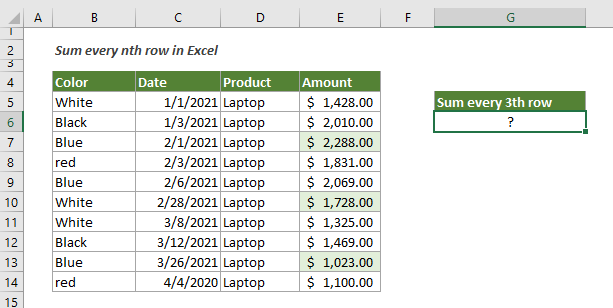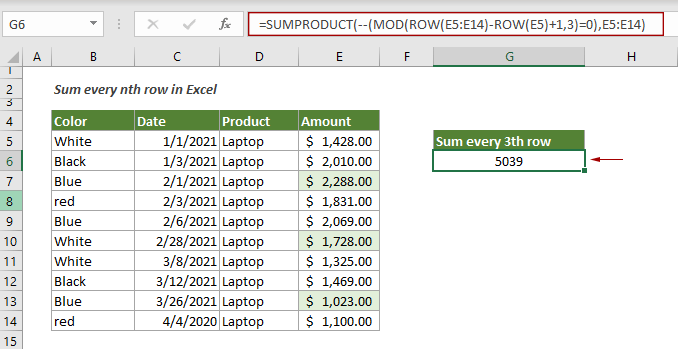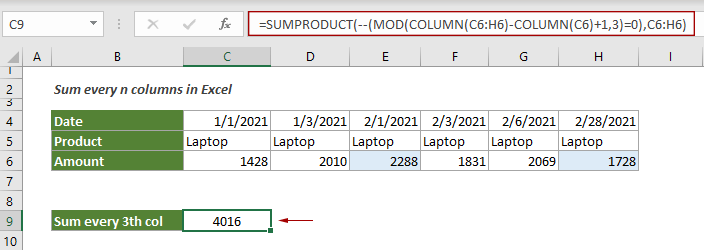## Sum every nth row or column in a range in Excel

This tutorial is going to show you how to create a formula based on the SUMPRODUCT, MOD and COLUMN functions to sum every nth row or column in a range in Excel.#### How to sum every nth row or column in a given range in Excel?

As shown above, to sum every third row in the Amount column, you can apply the below formula.

Generic Formulas

=SUMPRODUCT(--(MOD(ROW(value_range)-ROW(first_cell)+1,n)=0),value_range)

Arguments

Value_range: The range of values to sum;
First_cell: The first cell in the value_range;
N: A number that represents every nth row used to find the sum.

How to use this formula?

1. Select a blank cell.

2. Copy or enter the below formula into it and press the Enter key to get the result.

=SUMPRODUCT(--(MOD(ROW(E5:E14)-ROW(E5)+1,3)=0),E5:E14)Notes: To sum every nth column in a certain row range, you just need to replace all ROWs in the formula with COLUMNs to get it done as follows.

=SUMPRODUCT(--(MOD(COLUMN(C6:H6)-COLUMN(C6)+1,3)=0),C6:H6)Explanation of the formula

=SUMPRODUCT(--(MOD(ROW(E5:E14)-ROW(E5)+1,3)=0),E5:E14)

ROW(E5:E14)-ROW(E5)+1:
> ROW(E5:E14): The ROW function gets the relative row numbers for the range E5:E14, and returns an array like this: {5;6;7;8;9;10;11;12;13;14};
> ROW(E5): The ROW function here returns the row number of 5 for cell E5;
> {5;6;7;8;9;10;11;12;13;14}-{5}+1: Then each number in the array subtracts number 5 and then adds 1 to get a new array: {1;2;3;4;5;6;7;8;9;10}.
MOD(({1;2;3;4;5;6;7;8;9;10},3): The MOD function returns the remainder of each number in the array divided by 3 (here the number 3 is the value for N): {1;2;0;1;2;0;1;2;0;1}; In this array, 0s represent row 3, 6, 9, etc.
SUMPRODUCT(--({1;2;0;1;2;0;1;2;0;1}=0),E5:E14):
> --({1;2;0;1;2;0;1;2;0;1}=0): Here each remainder compares to 0 to force a TRUE or FALSE array like this {FALS;FALSE;TRUE;FALS;FALSE;TRUE;FALS;FALSE;TRUE;FALSE}; Then the two minus signs convert the “TRUE” and “FALSE” into 1s and 0s: {0;0;1;0;0;1;0;0;1;0};
> SUMPRODUCT({0;0;1;0;0;1;0;0;1;0},E5:E14)=SUMPRODUCT({0;0;1;0;0;1;0;0;1;0},{1428;2010;2288;1831;2069;1728;1325;1469;1023;1100}): Each pair of entries in the arrays is multiplied together and returns {0;0;2288;0;0;1728;0;0;1023;0}, and then the SUMPRODUCT function adds up all numbers in the array and returns the final result as 5039.

#### Related functions

Excel SUMPRODUCT function
The Excel SUMPRODUCT function can be used to multiply two or more columns or arrays together, and then get the sum of products.

Excel MOD function
The Excel MOD function returns the remainder after number is divided by divisor.

Excel ROW function
The Excel ROW function returns the row number of a reference.

Excel COLUMN function
The Excel COLUMNS function returns the number of column which formula appears or returns the column number of given reference.

#### Related formulas

Sum values by month (with or without year)
This tutorial demonstrates two formulas in detail to help summing values by certain month and year or summing values by certain month ignore year in Excel.

Sum values by the week of a given date
This tutorial talks about how to sum values by the week in which a given date falls

Sum values by weeknum or weekday
This tutorial demonstrates formulas step-by-step to help you sum values in a certain range based on the specified week number or weekday in Excel.

Sum every n rows or columns in a range
This tutorial explains two formulas based on the SUM and OFFSET functions to sum every n rows or columns in a specified range in Excel.

### The Best Office Productivity Tools

#### Kutools for Excel - Helps You To Stand Out From Crowd

 Popular Features: Find, Highlight or Identify Duplicates  |  Delete Blank Rows  |  Combine Columns or Cells without Losing Data  |  Round without Formula ... Super VLookup: Multiple Criteria  |  Multiple Value  |  Across Multi-Sheets  |  Fuzzy Lookup... Adv. Drop-down List: Easy Drop Down List  |  Dependent Drop Down List  |  Multi-select Drop Down List... Column Manager: Add a Specific Number of Columns  |  Move Columns  |  Toggle Visibility Status of Hidden Columns  |  Compare Columns to Select Same & Different Cells ... Featured Features: Grid Focus  |  Design View  |  Big Formula Bar  |  Workbook & Sheet Manager | Resource Library (Auto Text)  |  Date Picker  |  Combine Worksheets  |  Encrypt/Decrypt Cells  |  Send Emails by List  |  Super Filter  |  Special Filter (filter bold/italic/strikethrough...) ... Top 15 Toolsets:  12 Text Tools (Add Text, Remove Characters ...)  |  50+ Chart Types (Gantt Chart ...)  |  40+ Practical Formulas (Calculate age based on birthday ...)  |  19 Insertion Tools (Insert QR Code, Insert Picture from Path ...)  |  12 Conversion Tools (Numbers to Words, Currency Conversion ...)  |  7 Merge & Split Tools (Advanced Combine Rows, Split Excel Cells ...)  |  ... and more

Kutools for Excel Boasts Over 300 Features, Ensuring That What You Need is Just A Click Away...#### Office Tab - Enable Tabbed Reading and Editing in Microsoft Office (include Excel)

• One second to switch between dozens of open documents!
• Reduce hundreds of mouse clicks for you every day, say goodbye to mouse hand.
• Increases your productivity by 50% when viewing and editing multiple documents.
• Brings Efficient Tabs to Office (include Excel), Just Like Chrome, Edge and Firefox.